Next: The principle of equal Up: Statistical mechanics Previous: Introduction

## Specification of the state of a many particle system

How do we determine the state of a many particle system? Well, let us, first of all, consider the simplest possible many particle system, which consists of a single spinless particle moving classically in one dimension. Assuming that we know the particle's equation of motion, the state of the system is fully specified once we simultaneously measure the particle's position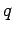and momentum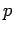. In principle, if we knowandthen we can calculate the state of the system at all subsequent times using the equation of motion. In practice, it is impossible to specifyandexactly, since there is always an intrinsic error in any experimental measurement.

Consider the time evolution ofand. This can be visualized by plotting the point (,) in the-plane. This plane is generally known as phase-space. In general, the point (,) will trace out some very complicated pattern in phase-space. Suppose that we divide phase-space into rectangular cells of uniform dimensions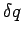and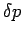. Here,is the intrinsic error in the position measurement, andthe intrinsic error in the momentum measurement. The area'' of each cell is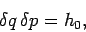(85)

whereis a small constant having the dimensions of angular momentum. The coordinatesandcan now be conveniently specified by indicating the cell in phase-space into which they plot at any given time. This procedure automatically ensures that we do not attempt to specifyandto an accuracy greater than our experimental error, which would clearly be pointless.

Let us now consider a single spinless particle moving in three dimensions. In order to specify the state of the system we now need to know three-pairs: i.e.,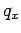-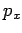,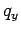-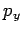, and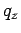-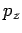. Incidentally, the number of-pairs needed to specify the state of the system is usually called the number of degrees of freedom of the system. Thus, a single particle moving in one dimension constitutes a one degree of freedom system, whereas a single particle moving in three dimensions constitutes a three degree of freedom system.

Consider the time evolution of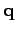and, where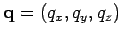, etc. This can be visualized by plotting the point (,) in the six dimensional-phase-space. Suppose that we divide the-plane into rectangular cells of uniform dimensionsand, and do likewise for the-and-planes. Here,andare again the intrinsic errors in our measurements of position and momentum, respectively. This is equivalent to dividing phase-space up into regular six dimensional cells of volume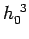. The coordinatesandcan now be conveniently specified by indicating the cell in phase-space into which they plot at any given time. Again, this procedure automatically ensures that we do not attempt to specifyandto an accuracy greater than our experimental error.

Finally, let us consider a system consisting ofspinless particles moving classically in three dimensions. In order to specify the state of the system, we need to specify a large number of-pairs. The requisite number is simply the number of degrees of freedom,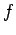. For the present case,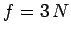. Thus, phase-space (i.e., the space of all the-pairs) now possesses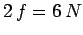dimensions. Consider a particular pair of conjugate coordinates,and. As before, we divide the-plane into rectangular cells of uniform dimensionsand. This is equivalent to dividing phase-space into regular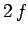dimensional cells of volume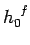. The state of the system is specified by indicating which cell it occupies in phase-space at any given time.

In principle, we can specify the state of the system to arbitrary accuracy by taking the limit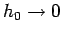. In reality, we know from quantum mechanics that it is impossible to simultaneously measure a coordinateand its conjugate momentumto greater accuracy than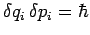. This implies that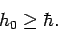(86)

In other words, the uncertainty principle sets a lower limit on how finely we can chop up classical phase-space.

In quantum mechanics we can specify the state of the system by giving its wave-function at time,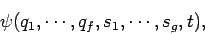(87)

whereis the number of translational degrees of freedom, andthe number of internal (e.g., spin) degrees of freedom. For instance, if the system consists ofspin-one-half particles then there will be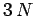translational degrees of freedom, andspin degrees of freedom (i.e., the spin of each particle can either point up or down along the-axis). Alternatively, if the system is in a stationary state (i.e., an eigenstate of the Hamiltonian) then we can just specify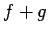quantum numbers. Either way, the future time evolution of the wave-function is fully determined by Schrödinger's equation.

In reality, this approach does not work because the Hamiltonian of the system is only known approximately. Typically, we are dealing with a system consisting of many weakly interacting particles. We usually know the Hamiltonian for completely non-interacting particles, but the component of the Hamiltonian associated with particle interactions is either impossibly complicated, or not very well known (often, it is both!). We can define approximate stationary eigenstates using the Hamiltonian for non-interacting particles. The state of the system is then specified by the quantum numbers identifying these eigenstates. In the absence of particle interactions, if the system starts off in a stationary state then it stays in that state for ever, so its quantum numbers never change. The interactions allow the system to make transitions between different stationary'' states, causing its quantum numbers to change in time.Next: The principle of equal Up: Statistical mechanics Previous: Introduction
Richard Fitzpatrick 2006-02-02